Rotations and Animations (Next Section)Chaos in the Classroom (Cover Page)Fractal Dimension (Previous Section)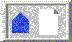BU Math Home Page

# Changing the rules in the chaos game

The chaos game provides a wonderful opportunity for students to develop their geometric insight as well as their understanding of the geometry of linear transformations. As we have seen, if we choose 3 points on the vertices of an equilateral triangle and play the chaos game, moving half the distance toward the appropriate vertex at each stage, then the Sierpinski triangle S results. Note that these numbers are reflected in the geometry of S, for this figure consists of 3 self-similar copies of S, each 1/2 the size of the S (or, as we said earlier, with magnification factor 2).

We really should have called S the Sierpinski equilateral triangle, since there are other Sierpinski triangles. For, as shown in Figure 9, if we begin with vertices on a right triangle or on a triangle with an obtuse angle, different fractals result. However, each of these images consists of 3 self-similar copies, each with magnification factor 2.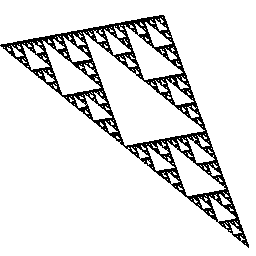Figure 9: Other Sierpinski triangles

Now let's vary the rules of the chaos game. Suppose we again start with 3 points at the vertices of an equilateral triangle. We will again move toward these vertices depending upon the roll of a die. This time, if the lower 2 vertices are chosen, we again move 1/2 the distance toward them. But if the top vertex is chosen, we move 2/3 of the way toward that vertex. A better way to say this (for reasons that will be clear in a moment) is: We compress the distance from the point to the top vertex by 1/3. Equivalently, we move our point on a straight line to the top vertex so that the new distance is 1/3 of the old. If we play the chaos game with these rules, a very different image results (figure 10A). However, note that this image consists of three self-similar pieces, two of which are exactly 1/2 the size of the entire image, while the other is 1/3 the size. Again, the number of basic copies is equal to the number of vertices we started with, and the magnification factors at each vertex correspond as well.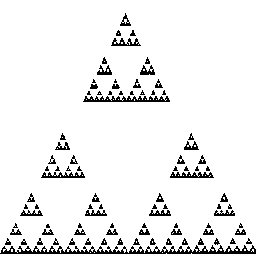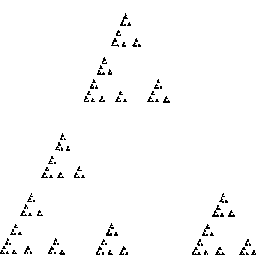Figure 10: The result of playing the chaos game with 3 vertices and magnification factors 2, 2, and 3, and then with magnification factors 2, 3, 4

If we further refine the rules of the chaos game, we see that we can still read off the original rules we used to play the game from the geometry of the resulting figure. For example, in figure 10B, we used 3 vertices and magnificat ion factors 2, 3 and 4 to create the image (that is, we moved 1/2 the distance toward one vertex, 1/3 the distance toward another, and 1/4 the distance to the final vertex).

We may also vary the number of vertices used. For example, to produce figure 11A, we chose 6 points on the vertices of a regular hexagon and moved 1/3 the distance toward the appropriate vertex at each roll of the die. Note that this is a ``natural'' chaos game in the sense that we can number the vertices 1-6 and use the number that comes up at each roll to determine the vertex to move toward. We call this image the Sierpinski hexagon; note that it consists of 6 self-similar pieces, each with magnification factor 3. So the fractal dimension of this image is N^2.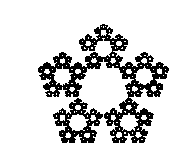Figure 11: The Sierpinski hexagon and pentagon

There is another famous fractal buried inside the Sierpinski hexagon. Note that the inner boundary of this figure is the well known Koch snowflake curve (see Devaney, 1990).

If we choose 5 vertices on a regular pentagon, and compress toward the vertices by a factor of 3/8 at each roll of a 5-sided die, the image in figure 11B results---a Sierpinski pentagon. Again we find 5 self-similar copies, each 1/3 the size of the original.

At this point it is interesting to guess what figure results when we play the chaos game with points on the vertices of a square and a magnification factor of 2. Most people expect a ``Sierpinski square.'' Wrong! The points fill out the entire square when the chaos game is played with these rules. But that is no surprise: After all, a square is a self-similar image that may be broken into 4 self-similar pieces, each with magnification factor 2. So we can again read off the rules of the chaos game from the resulting figure.Rotations (Next Section)Chaos in the Classroom (Cover Page)Fractal Dimension (Previous Section)BU Math Home Page

Robert L. Devaney
Sun Apr 2 14:31:18 EDT 1995